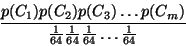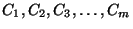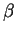# Finding coding regions in DNA sequences

Unequal usage of codons in the coding regions appears to be a universal feature of the genomes across the phylogenetic spectra. This bias obeys mainly to (i) the uneven usage of the amino acids in the existing proteins and (ii) the uneven usage of synonymous codons. The bias in the usage of the synonymous codons correlates with the abundance of the corresponding tRNAs. The correlation is particularly strong for highly expressed genes. Codon usage is specific of the taxonomic group, and there exist correlation between taxonomic divergence and similarity of codon usage.

By comparing the frequency of codons in a region of an species genome read in a given frame with the typical frequency of codons in the species genes, it is possible to estimate a likelihood of the region coding for a protein in such a frame. Regions in which codons are used with frequencies similar to the typical species codon frequencies are likely to code for genes (exons) while regions which codons distribution is uniform -- 1/64 (0.015625) -- could be considered as non-coding regions (introns).

 The Human Codon Usage Table Gly GGG 17.08 0.23 Arg AGG 12.09 0.22 Trp TGG 14.74 1.00 Arg CGG 10.40 0.19 Gly GGA 19.31 0.26 Arg AGA 11.73 0.21 End TGA 2.64 0.61 Arg CGA 5.63 0.10 Gly GGT 13.66 0.18 Ser AGT 10.18 0.14 Cys TGT 9.99 0.42 Arg CGT 5.16 0.09 Gly GGC 24.94 0.33 Ser AGC 18.54 0.25 Cys TGC 13.86 0.58 Arg CGC 10.82 0.19 Glu GAG 38.82 0.59 Lys AAG 33.79 0.60 End TAG 0.73 0.17 Gln CAG 32.95 0.73 Glu GAA 27.51 0.41 Lys AAA 22.32 0.40 End TAA 0.95 0.22 Gln CAA 11.94 0.27 Asp GAT 21.45 0.44 Asn AAT 16.43 0.44 Tyr TAT 11.80 0.42 His CAT 9.56 0.41 Asp GAC 27.06 0.56 Asn AAC 21.30 0.56 Tyr TAC 16.48 0.58 His CAC 14.00 0.59 Val GTG 28.60 0.48 Met ATG 21.86 1.00 Leu TTG 11.43 0.12 Leu CTG 39.93 0.43 Val GTA 6.09 0.10 Ile ATA 6.05 0.14 Leu TTA 5.55 0.06 Leu CTA 6.42 0.07 Val GTT 10.30 0.17 Ile ATT 15.03 0.35 Phe TTT 15.36 0.43 Leu CTT 11.24 0.12 Val GTC 15.01 0.25 Ile ATC 22.47 0.52 Phe TTC 20.72 0.57 Leu CTC 19.14 0.20 Ala GCG 7.27 0.10 Thr ACG 6.80 0.12 Ser TCG 4.38 0.06 Pro CCG 7.02 0.11 Ala GCA 15.50 0.22 Thr ACA 15.04 0.27 Ser TCA 10.96 0.15 Pro CCA 17.11 0.27 Ala GCT 20.23 0.28 Thr ACT 13.24 0.23 Ser TCT 13.51 0.18 Pro CCT 18.03 0.29 Ala GCC 28.43 0.40 Thr ACC 21.52 0.38 Ser TCC 17.37 0.23 Pro CCC 20.51 0.33

Let S a sequence of DNA, the higher the number of codons in S matching the more frequent codons in the table, the higher the coding potential of the sequence S (the probability to be encoding a protein). This value can be computed as:whereare the codons as.

This expression is known as likelihood ratio and it is usually computed in logarithmics terms to reduce the magnitude of the numbers (log-likelihood ratio).

1. Scan a sequence, computing the protein coding potential:

Here, you can find the human codon usage table in a more manageable format (tabular format).

 We are going to write a program in order to measure the coding potential of a sequence in Fasta format taking into account a given table of codon usage.

• Copy the following code into a file that we will call dnaloglkh.pl:

```#!/usr/bin/perl -w

use strict;

# if the number of input arguments is lower than 2
# return a message showing the error

if (scalar(@ARGV) < 2) {
print "dnaloglkh.pl codontable DNAsequence\n";
exit(1);
}

# create two vars contaning the filenames

my \$filecodontable = \$ARGV;
my \$filesequence = \$ARGV;

# open the first file: table of codon usage frequencies

if (!open(PROPCODONS,"< \$filecodontable")) {
print "dnaloglkh.pl: the file \$filecodontable can not be opened\n";
exit(1);
}

# load the frequencies of codon usage into the hash table %pcodons
# this hash is indexed by a triplet of nucleotides or codon

my %pcodons;
my (\$codon,\$freq);
my \$line;

# for each line in the file (tableofcodons) do

while (\$line = <PROPCODONS>) {

# extract the two values or columns with a regular expression
# A group of letters and a decimal number

(\$codon,\$freq) = (\$line =~ m/(\w+) (\d+\.\d+)/);

# load the hash table with the frequency of the current codon
********* FILL IN 1 *********
}

close(PROPCODONS);
```

This sketch of program verifies that the user has provided 2 arguments in the input. The first one is the name of the file containing the table of codon usage. Once, the file has been opened, the content of the file (pairs codon,probability) will be save into a hash table called %pcodons. Please, complete the program with a single instruction to load the current probablity into the hash indexed with the current codon.

• After the previous fragment of program, copy this sketch:

```##########################################################
# open the DNA sequence (second file)

if (!open(SEQUENCE,"< \$filesequence")) {
print "dnaloglkh.pl: the file \$filesequence can not be opened\n";
exit(1);
}

# FASTA format:
# >header containing a description of the sequence
# AGACTGACTTAC
# AGACTGACTTAC
# AGACTGAC

my @seql = <SEQUENCE>;  # load the complete sequence into an array
my \$seq  = "";          # use a var to save the whole sequence inside

close(SEQUENCE);

# concat every segment (line) in the array into the var \$seq

my \$i=0;

while (\$i < scalar(@seql)) {
chomp(\$seql[\$i]);
\$seq = ********* FILL IN 2 *********
\$i = \$i + 1;
}

\$seq = "\U\$seq";
```

• Right now, the variable \$seq contains the sequence of DNA. Finally, the log-likelihood ratio must be computed, codon by codon. Add the last part of the program to complete it:
```# we are going to use the array @codons to store all of the codons in
# the input DNA sequence. To split the sequence in segments of length
# three, a regular expression will be used searching for all of the
# possible groups of three symbols (option g)

my @codons = (\$seq =~ m/.../g);

# the likelihood ratio is for a current triplet, the ratio between
# the probability computed from the table of codon frequencies divided
# by the random probability (uniform), that is, 1/64. After this,
# the log must be applied to obtain the log-likelihood ratio.
# It is recommended the use of logs to manage calculations involving
# small numbers between 0 and 1. Log of the product is equal to the
# the sum of logs and the log of the division is the substraction of logs

my \$r = 0.0;

\$i=0;
while (\$i < scalar(@codons)) {
********* FILL IN 3 *********
\$i = \$i + 1;
}
printf "%.2f\n",\$r;
```

If you can not suceed, you can take a look at the complete program, here.

• To test the program, save this two files in your current working directory: exon.fa and intron.fa. Both files contain the sequence of a real exon and intron, respectively. Extremely different values of coding potential should be obtained with the two sequences: high values (positive) for coding protein sequences and low values (zero or even negative) for intronic regions.

2. Compute the protein coding potential in the three reading frames:

Unfortunately, as you have seen the log-likelihood value computed for both cases is very similar so that we can not distinguish among coding and non coding regions. As you know, a DNA sequence can be translated into a series of amino acids using three different reading frames and we are probably using the wrong frame right now.

 We will modify the previous program to read an extra argument defining the reading frame (0,1,2) that will be used to measure the coding potential in the input sequence.

Let us suppose that there is third argument (the reading frame) that will be saved into the variable \$ARGV. Just before converting the sequence into a list of codons (before the line my @codons = (\$seq =~ m/.../g);), we can delete zero, one or two characters from the beginning of the sequence with the command substr().

• Insert this line over there:
```\$seq = substr(\$seq,\$ARGV);
```
The function substr(s,p,l) extracts a subsequence of "s", starting from the point "p" that contains "l" symbols. If the third parameter is omitted, then the substring will be constructed from "p" to the end of the sequence "s".

• Run your new program with the frame 0:
```\$ ./dnaloglkh.pl propcodons.txt exon.fa 0
```
• Try the other reading frames to realize that the exon can be detected in the frame 1.

3. Compute the protein coding potential using sliding windows in the three reading frames at the same time:

In practice, the problem is not to determine the likelihood that a given sequence is coding or not, but to locate the (usually small) coding regions within large genomic sequences. The typical procedure is to compute the value of a coding statistic in successive (overlapped) sliding windows, and record the value of the statistic for each of the windows. This generates a profile along the sequence in which peaks may point to the coding regions and valleys to the non-coding ones.

• Take a look at the following program and save it as dnaloglkh2.pl. As you can see, it is the same program you have completed before but the part of the program that computes the log-likelihood value of the sequence in a given frame has been encapsulated into a function called compute_loglkh.
```#!/usr/bin/perl -w

use strict;

# if the number of input arguments is lower than 3
# return a message showing the error

if (scalar(@ARGV) < 3) {
print "dnaloglkh.pl codontable DNAsequence frame\n";
exit(1);
}

# Load the arguments into three vars
my \$filecodontable = \$ARGV;
my \$filesequence = \$ARGV;
my \$frame = \$ARGV;

# open the first file: table of codon usage frequencies

if (!open(PROPCODONS,"< \$filecodontable")) {
print "dnaloglkh.pl: the file \$filecodontable can not be opened\n";
exit(1);
}

# load the frequencies of codon usage into the hash table %pcodons
# this hash is indexed by a triplet of nucleotides or codon

my %pcodons;
my (\$codon,\$freq);
my \$line;

# for each line in the file (tableofcodons) do

while (\$line = <PROPCODONS>) {

# extract the two values or columns with a regular expression
# A group of letters and a decimal number

(\$codon,\$freq) = (\$line =~ m/(\w+) (\d+\.\d+)/);

\$pcodons{\$codon}=\$freq;
}

close(PROPCODONS);

##########################################################
# open the DNA sequence (second file)

if (!open(SEQUENCE,"< \$filesequence")) {
print "dnaloglkh.pl: the file \$filesequence can not be opened\n";
exit(1);
}

# FASTA format:
# >header containing a description of the sequence
# AGACTGACTTAC
# AGACTGACTTAC
# AGACTGAC

my @seql = <SEQUENCE>;  # load the complete sequence into an array
my \$seq  = "";          # use a var to save the whole sequence inside

close(SEQUENCE);

# concat every segment (line) in the array into the var \$seq

my \$i=0;

while (\$i < scalar(@seql)) {
chomp(\$seql[\$i]);
\$seq = \$seq . \$seql[\$i];
\$i = \$i + 1;
}

\$seq = "\U\$seq";

# using the function to define the operation of log-likelihood

printf "%.2f\n",compute_loglkh(\$seq,\$ARGV);

##############################################################
##############################################################
#############            FUNCTIONS        ####################
##############################################################
##############################################################

# NAME: compute_loglkh
# GOAL: compute the log-likelihood of a sequence using
# PARAMS: first  - DNA sequence
#         second - reading frame =  {0,1,2}
# RETURNS: the log-likelihood ratio (coding vs non-coding region)

sub compute_loglkh {
my \$seq   = \$_;
my \$frame = \$_;

# the function substr(s,p,l) extract a subsequence of "s" from
# the position "p" having "l" symbols (if omitted, extract from that
# point to the end

\$seq = substr(\$seq,\$frame);

# we are going to use the array @codons to store all of the codons in
# the input DNA sequence. To split the sequence in segments of length
# three, a regular expression will be used searching for all of the
# possible groups of three symbols (option g)

my @codons = (\$seq =~ m/.../g);

# the likelihood ratio is for a current triplet, the ratio between
# the probability computed from the table of codon frequencies divided
# by the random probability (uniform), that is, 1/64. After this,
# the log must be applied to obtain the log-likelihood ratio.
# It is recommended the use of logs to manage calculations involving
# small numbers between 0 and 1. Log of the product is equal to the
# the sum of logs and the log of the division is the substraction of logs

my \$r = 0.0;
my \$i=0;

while (\$i < scalar(@codons)) {

\$r = \$r + log(\$pcodons{\$codons[\$i]}) - log(1/64);

\$i = \$i + 1;

}

return \$r;
}
```

 We will add some commands to compute the coding potential in the three reading frames on series of windows or consecutive segments of DNA.

In order to find a coding region (exon) in a long sequence, we should compute the likelihood ratio in separate windows of a given length. Once the value has been obtained for the three frames, the window must be shifted a set of fixed positions (overlap).

• Copy the program dnaloglkh2.pl into a new one that will be called dnaloglkh3.pl. Then modify the program dnaloglkh3.pl: Insert the following code at the beginning to read four arguments from the input: codon usage table, DNA sequence, length of the window and length of the shift between old and new windows.

```#!/usr/bin/perl -w

use strict;

# if the number of input arguments is lower than 4
# return a message showing the error

if (scalar(@ARGV) < 4) {
print "dnaloglkh.pl codontable DNAsequence Lwindow Loverlap\n";
exit(1);
}

# Load the arguments into four vars
my \$filecodontable = \$ARGV;
my \$filesequence = \$ARGV;

# Length and overlap of the window to scan the sequence
my \$lengthwindow = \$ARGV;
my \$overlap = \$ARGV;
```
• Now, change the last action (printf) before the definition of the functions with the following iterative composition:

```# Scan the three possible frames for every window

\$i = 0;
while (\$i <= length(\$seq) - \$lengthwindow) {

# extract the segment of sequence corresponding to that window
# the function substr(s,p,l) extract a subsequence of "s" from
# the position "p" having "l" symbols

my \$seqwindow = substr(\$seq,** FILL IN 4 **,** FILL IN 5 **);

# compute the log-likelihood ratio for every reading frame

my \$frame0 = ********* FILL IN 6 *********
my \$frame1 = ********* FILL IN 7 *********
my \$frame2 = ********* FILL IN 8 *********

# take the maximum value. Display the value and the position

my \$maximum = max(\$frame0,\$frame1,\$frame2);

printf "%d %.5f\n",\$i,\$maximum;

# increase \$i with the \$overlap value

\$i = \$i + \$overlap;

}
```
• Finally, copy the definition of the function max() at the end of the program.

```# NAME: max
# GOAL: compute the maximum value in a list of N values
# PARAMS: a list of values
# RETURNS: the maximum value in the list

sub max {

my \$i = 1;
my \$max = \$_;

while (\$i < scalar(@_)) {

if (\$_[\$i] > \$max) {
\$max = \$_[\$i];
}

\$i = \$i + 1;
}

return \$max;
}
```

• Run the program on the sequence hs.fa that encodes the three exons of the human-globin gene
```\$ ./dnaloglkh3.pl propcodons.txt hs.fa 120 10 > cu.dat
```
The file cu.dat contains the log-likelihood ratio computed for every 120 bps-window, shifting from 10 to 10 bps in the sequence HS, according to the table of human codon usage.

To analyze this information, we can visualize the results in a graphical way using the program gnuplot:

• Type in the shell the command:
```\$ gnuplot
```
• Then, copy the next command:
```gnuplot> plot 'cu.dat' with impulses
```
to obtain a plot like this:• On this plot, it is easy to identify the three regions whose coding potential is positive. Check that those regions are the three real exons that were experimentally verified:

``` 187 - 278
409 - 631
1482 - 1610
```